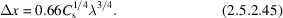International
Tables for
Crystallography
Volume B
Reciprocal space
Edited by U. Shmueli

International Tables for Crystallography (2010). Vol. B, ch. 2.5, pp. 306-307   | 1 | 2 |

## Section 2.5.2.9. Image resolution

J. M. Cowleya

#### 2.5.2.9. Image resolution

| top | pdf |

• (1) Definition and measurement. The resolution' of an electron microscope or, more correctly, the least resolvable distance', is usually defined by reference to the transfer function for the coherent imaging of a weak phase object under the Scherzer optimum defocus condition (2.5.2.44). The resolution figure is taken as the inverse of the U value for whichfirst crosses the axis and is given, as in (2.5.2.45), byIt is assumed that an objective aperture is used to eliminate the contribution to the image for U values greater than the first zero crossing, since for these contributions the relative phases are distorted by the rapid oscillations ofand the corresponding detail of the image is not directly interpretable in terms of the projection of the potential distribution of the object.

The resolution of the microscope in practice may be limited by the incoherent factors which have the effect of multiplying the WPOA transfer function by envelope functions as in (2.5.2.49).

The resolution, as defined above, and the effects of the envelope functions may be determined by Fourier transform of the image of a suitable thin, weakly scattering amorphous specimen. The Fourier-transform operation may be carried out by use of an optical diffractometer. A more satisfactory practice is to digitize the image directly by use of a two-dimensional detector system in the microscope or from a photographic recording, and perform the Fourier transform numerically.

For the optical diffractometer method, the intensity distribution obtained is given from (2.5.2.43)as a radially symmetric function of U,whereis the product of the envelope functions.

In deriving (2.5.2.54)it has been assumed that:

 (a) the WPOA applies; (b) the optical transmission function of the photographic record is linearly related to the image intensity,; (c) the diffraction intensityis a radially symmetric, smoothly varying function such as is normally produced by a sufficiently large area of the image of an amorphous material; (d) there is no astigmatism present and no drift of the specimen; either of these factors would remove the radial symmetry. From the form of (2.5.2.54)and a preknowledge of, the zero crossings ofand the form ofmay be deduced. Analysis of a through-focus series of images provides more complete and reliable information.

• (2) Detail on a scale much smaller than the resolution of the electron microscope, as defined above, is commonly seen in electron micrographs, especially for crystalline samples. For example, lattice fringes, having the periodicity of the crystal lattice planes, with spacings as small as 0.6 Å in one direction, have been observed using a microscope having a resolution of about 2.5 Å (Matsuda et al., 1978), and two-dimensionally periodic images showing detail on the scale of 0.5 to 1 Å have been observed with a similar microscope (Hashimoto et al., 1977).

Such observations are possible because

 (a) for periodic objects the diffraction amplitudein (2.5.2.31)is a set of delta functions which may be multiplied by the corresponding values of the transfer function that will allow strong interference effects between the diffracted beams and the zero beam, or between different diffracted beams; (b) the envelope functions for the WPOA, arising from incoherent imaging effects, do not apply for strongly scattering crystals; the more general expression (2.5.2.36)provides that the incoherent imaging factors will have much less effect on the interference of some sets of diffracted beams. The observation of finely spaced lattice fringes provides a measure of some important factors affecting the microscope performance, such as the presence of mechanical vibrations, electrical interference or thermal drift of the specimen. A measure of the fineness of the detail observable in this type of image may therefore be taken as a measure of `instrumental resolution'.

### References

Hashimoto, H., Endoh, H., Tanji, T., Ono, A. & Watanabe, E. (1977). Direct observation of fine structure within images of atoms in crystals by transmission electron microscopy. J. Phys. Soc. Jpn, 42, 1073–1074.
Matsuda, T., Tonomura, A. & Komada, T. (1978). Observation of lattice images with a field emission electron microscope. Jpn. J. Appl. Phys. 17, 2073–2074.